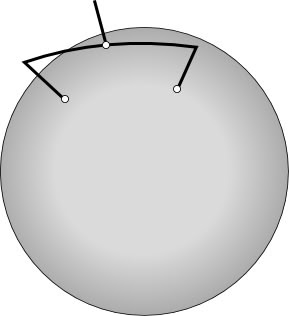Thursday, June 07, 2007

Earth not flat - official

Oops. One big problem with drawing trees in Google Earth is that the Earth, sadly, is not flat. This means that widely distributed clades cause problems if I draw straight lines between nodes in the tree. For geographically limited clades (such as the Hawaiian kaytidids shown earlier) this is not really a problem. But for something like plethodontid salamanders (data from TreeBASE study S1139, see doi:10.1073/pnas.0405785101), this is an issue.

One approach is to draw the tree using straight lines connecting nodes, and elevate the tree sufficiently high above the globe so that the lines don't intersect the globe. This is the approach taken by Bill Piel in his server. However, I want to scale the trees by branch length, and hence draw them as phylograms. The screenshot below should make this clearer. Note the salamander Hydromantes italicus in Europe (misspelt in TreeBASE as Hydromantes italucus, but that's another story).(You can grab the KML file here).

This means that for each internal node in the tree I need to draw a line parallel to the Earth's surface. Oddly, Google Earth seems not to have an easy way to do this. So, we have to do this ourselves. Essentially this requires computing great circles. I managed to find Ed Williams' Aviation Formulary, which gives the necessary equations. The great circle distance d between two points with coordinates {lat1,lon1} and {lat2,lon2} is given by:
`d=acos(sin(lat1)*sin(lat2)+cos(lat1)*cos(lat2)*cos(lon1-lon2))`

where the latitudes and longitudes are in radians rather than degrees. What we then need is a set of points along the great circle route between the two points. In the following equations f is the fractional distance along the route, where 0 is the first point and 1 is the second point:
`A=sin((1-f)*d)/sin(d)B=sin(f*d)/sin(d)x = A*cos(lat1)*cos(lon1) +  B*cos(lat2)*cos(lon2)y = A*cos(lat1)*sin(lon1) +  B*cos(lat2)*sin(lon2)z = A*sin(lat1)           +  B*sin(lat2)lat=atan2(z,sqrt(x^2+y^2))lon=atan2(y,x)`

So, to draw a tree for any internal node point 1 is the latitude and longitude of the left child, point 2 is the latitude and longitude of the right child, and we place the internal node at the midpoint of the great circle route (f = 0.5). This diagram shows the construction (based on the Astronomy Answers Great Circle page).To draw the line compute coordinates for f = 0.1, f = 0.2, etc. and join the dots.The data for the localities for the sequences is slightly tortuous to obtain. The original paper (doi:10.1073/pnas.0405785101) has a spreadsheet listing MVZ specimen numbers, which I then looked up using a Perl script to interrogate the MVZ DiGIR provider. I grabbed the NEXUS file from TreeBASE, built a quick neighbour joining tree, pruned off four taxa that had no geographic coordinates, then drew the tree in KML. Of course, in an ideal world all this should be easy (TreeBASE linked to sequences, which are linked to specimens, which are linked to geography), but for now just being able to make pictures like this is kinda fun.

Kehan Harman said...

Looks pretty in Google Maps as well: